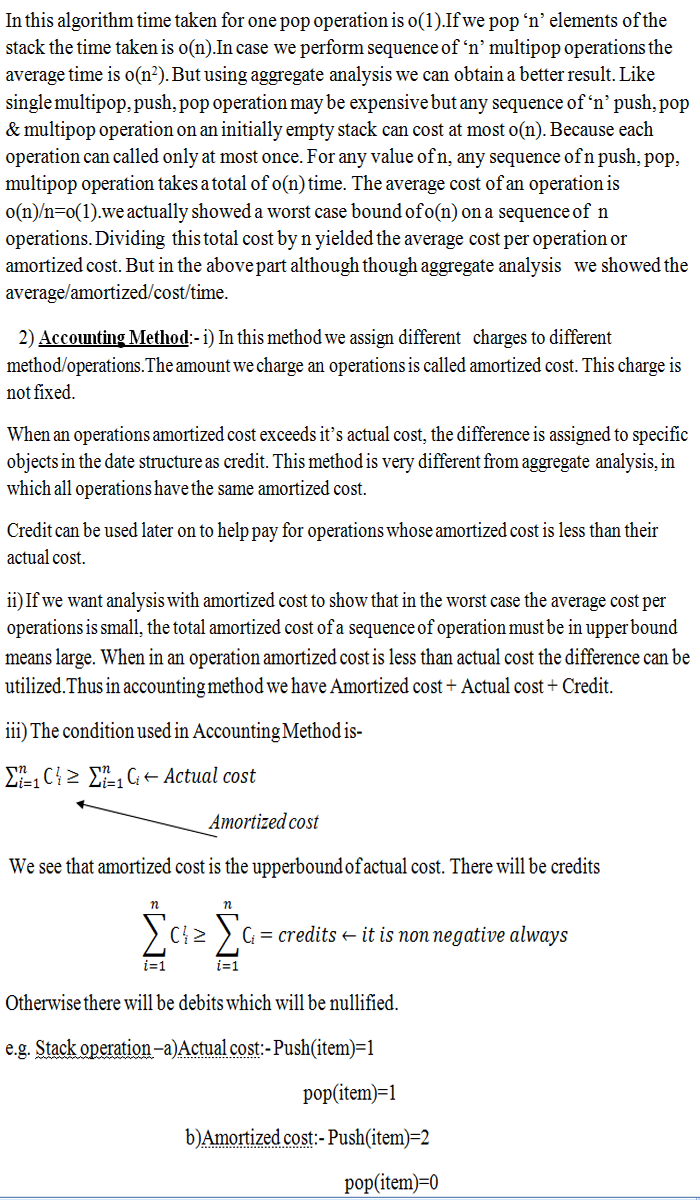## DESIGN AND ANALYSIS OF ALGORITHM

It's all about self learning.

Amortized Algorithm and Types of Amortized Algorithm
6 November, 2016

This means finding average running time per operation over a worst case sequence of operations.

This analysis shows that average cost/time of an operation is small even though cost of sum of the operation is large.

The difference between amortized cost/time & average case is that in amortized we are averaging over a sequence of inputs and operation and in the case of average we just averaging over various inputs.

It has basically three parts:-
a) Aggregate analysis
b) Accounting method
c) Potential method

Aggregate Analysis:- In this type of analysis, we have a sequence of ‘n’ operations and total worst case time taken by these operations is T(n).

But in worst case, the average cost or amortized cost, per operation is therefore T(n)/n. This amortized cost applies to each operation, even when there  are several type of operation in the sequence.

e.g.  Consider an example of stack operation which are  push and pop.The routines for them are:-
Push-
{

If the stack to p is not empty then
return(0)
else
push = item

}
T(n) = o(1)
Pop-
{
If stack is empty
return(0)
else
pop = item
}
T(n) = o(1)

Amortize time = (o(1)+o(1))/2 = o(1)

In case we have to pop ‘n’ element then amortized time is o(n).

e.g. Write a function of performing multiple pop operation.

Ans.  Algo:-
Algo multipop(st,k)  //where k is No. of element
{
{

While(st!=empty and k!=0)do
Pop(st);
K=k-1;

}
}In actual cost we have two operations push and pop. Each time when we execute these operation we have to call it string data in STACK.

In amortized cost we assign Push = 2 when we push the item into empty stack it’s value become Push = 1. Then we no need to assign the value to pop because the data stored in stack is prepayment for the cost of popping function.When we execute pop operation,we charge nothing. Thus amortized cost is an upper bound on the Total actual cost. Hence the amortized cost is still O(1).